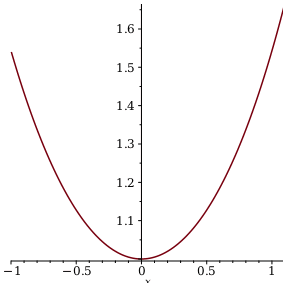The Student Statistics Package - Maple Help

Home : Support : Online Help : Applications and Example Worksheets : Statistics : The Student Statistics Package

The Student Statistics PackageDescription The Student[Statistics] subpackage is designed to help teachers present and students understand the basic material of a standard course in statistics.Getting Started

While any command in the package can be referred to using the long form, for example, Student[Statistics][NormalRandomVariable], it is often easier to load the package and then use the short form command names.

 > restart;
 > with(Student[Statistics]):Quantities

 > A := BinomialRandomVariable(5, 1/3):
 > Probability({A>=2, A<=4});
 $\frac{{130}}{{243}}$ (1)
 > Mean(A);
 $\frac{{5}}{{3}}$ (2)
 > Correlation(A, A^2);
 $\frac{{11}}{{1370}}\sqrt{{10}}\sqrt{{1370}}$ (3)
 > M := Matrix([[1,2,4,1],[2,4,1,2],[6,5,2,7],[1,2,0,9]]);> Variance(M);> L := [1, 2, 3, 1, 2, 3, 1, 2, 2, 2, 6, 2, 3, 4, 5, 2, 4]:
 > Mode(L);
 $\left\{{2}\right\}$ (4)
 > Percentile(L, 30);
 ${2}$ (5)Formulas

 > B := NormalRandomVariable(1,2):
 > Probability(B
 ${{∫}}_{{-}{\mathrm{∞}}}^{{x}}\frac{{1}}{{4}}\frac{\sqrt{{2}}{{ⅇ}}^{{-}\frac{{1}}{{8}}{\left({\mathrm{_t2}}{-}{1}\right)}^{{2}}}}{\sqrt{{\mathrm{π}}}}\phantom{\rule[-0.0ex]{0.3em}{0.0ex}}{ⅆ}{\mathrm{_t2}}$ (6)
 > CumulativeDistributionFunction(B, 3, output=plot);Hypothesis Testing

 > X := [6, 3, 2, 1, 9, 1, 2, 3, 3, 2]:
 > StandardDeviation(X);
 $\frac{{1}}{{15}}\sqrt{{1390}}$ (7)
 > ChiSquareSuitableModelTest(X, PoissonRandomVariable(3));
 Chi-Square Test for Suitable Probability Model ---------------------------------------------- Null Hypothesis: Sample was drawn from specified probability distribution Alt. Hypothesis: Sample was not drawn from specified probability distribution Bins:                    5 Degrees of freedom:      4 Distribution:            ChiSquare(4) Computed statistic:      2.20801 Computed pvalue:         0.697563 Critical value:          9.48772903678116 Result: [Accepted] There is no statistical evidence against the null hypothesis
 $\left[{\mathrm{hypothesis}}{=}{\mathrm{true}}{,}{\mathrm{criticalvalue}}{=}{9.48772903678116}{,}{\mathrm{distribution}}{=}{\mathrm{ChiSquare}}\left({4}\right){,}{\mathrm{pvalue}}{=}{0.697562873819393}{,}{\mathrm{statistic}}{=}{2.20801128786324}\right]$ (8)Interactive Exploration of Random Variables

The command ExploreRV takes an arbitrary statistical distribution and displays an interactive interface to explore its various parameters.

 > ExploreRV(NormalRandomVariable(mu, sigma));

Random Variables:

Parameters:

 ${\mathrm{mu___R4}}$${\mathrm{sigma___R4}}$Statistical Properties:

 MeanSupportMedianVarianceModeMoment Generating FunctionProbability Distribution FunctionCumulative Distribution FunctionExample 1

 > Distribution1 := BinomialRandomVariable(7,1/2):
 > Mean(Distribution1);
 $\frac{{7}}{{2}}$ (9)
 > StandardDeviation(Distribution1);
 $\frac{{1}}{{2}}\sqrt{{7}}$ (10)

To return a numeric value, add the numeric option.

 > StandardDeviation(Distribution1, numeric);
 ${1.322875656}$ (11)

Setting the output option to plot returns a plot demonstration.

 > ProbabilityFunction(Distribution1,x,output=plot);> CDF(Distribution1, 3, output = plot);To get the formula for computing a specific property of a distribution, specify the optional parameter inert or inert=true.

 > Probability(Distribution1 <= 4, inert);
 ${\sum }_{{\mathrm{_t3}}{=}{0}}^{{4}}\phantom{\rule[-0.0ex]{5.0px}{0.0ex}}\left({{}\begin{array}{cc}{0}& {\mathrm{_t3}}{<}{0}\\ {\mathrm{binomial}}\left({7}{,}{\mathrm{_t3}}\right){\left(\frac{{1}}{{2}}\right)}^{{\mathrm{_t3}}}{\left(\frac{{1}}{{2}}\right)}^{{7}{-}{\mathrm{_t3}}}& {\mathrm{otherwise}}\end{array}\right)$ (12)Example 2

To randomly generate a data sample from a known distribution with the specified sample size, use the Sample command.

 > Sample1 := Sample(ExponentialRandomVariable(5), 1000);> InterquartileRange(Sample1);
 ${5.21348341554859}$ (13)
 > Median(Sample1);
 ${3.06138615867682}$ (14)

Compare the data sample generated and the original distribution.

 > Sample(ExponentialRandomVariable(5), 1000, output = plot);Test if this sample follows the exponential distribution with parameter 5.

 > ChiSquareSuitableModelTest(Sample1, ExponentialRandomVariable(5));
 Chi-Square Test for Suitable Probability Model ---------------------------------------------- Null Hypothesis: Sample was drawn from specified probability distribution Alt. Hypothesis: Sample was not drawn from specified probability distribution Bins:                    32 Degrees of freedom:      31 Distribution:            ChiSquare(31) Computed statistic:      33.472 Computed pvalue:         0.348188 Critical value:          44.9853428040743 Result: [Accepted] There is no statistical evidence against the null hypothesis
 $\left[{\mathrm{hypothesis}}{=}{\mathrm{true}}{,}{\mathrm{criticalvalue}}{=}{44.9853428040742}{,}{\mathrm{distribution}}{=}{\mathrm{ChiSquare}}\left({31}\right){,}{\mathrm{pvalue}}{=}{0.348188188879230}{,}{\mathrm{statistic}}{=}{33.47200000}\right]$ (15)Example 3

Create a Matrix data sample:

 > Matrix1 := Matrix(5, 3, {(1, 1) = 1, (1, 2) = 2, (1, 3) = 3, (2, 1) = 2, (2, 2) = Pi, (2, 3) = 5, (3, 1) = 9, (3, 2) = 7, (3, 3) = 3, (4, 1) = 5, (4, 2) = 5, (4, 3) = 2, (5, 1) = 2, (5, 2) = 8, (5, 3) = 10}):

Computing the mean of the matrix returns the three list or Vector data samples stored in the corresponding columns.

 > Mean(Matrix1);To have both value and plot returned, specify the option output=both.

 > Value, Graph := InterquartileRange(Matrix1, output = both):
 > Value;> Graph;See Also